Close# Sound intensity

Auer Signal is a global leading manufacturer of signaling technology, operational in 70+ countries. The company produces stack lights, visual-, audible-, visual-audible and explosion proof signaling devices.

## What is sound intensity?

The intensity of sound is the subjective perception of sound pressure. Every person has a different perception of loudness, which means that loudness cannot be measured objectively. The acoustic sound intensity / audio intensity or acoustic signal is therefore relative. The increasing amplitude of the source and that of the vibrating surface causes the kinetic energy of the mass of air.

The sound intensity formula is defined mathematically as: sound intensity=acousticpower/normal area to the direction of propagation

Physically measurable, on the other hand, is the sound pressure, which is converted into sound level and further expressed in decibels (dB). The second measurable quantity is hertz, which measures the number of air pressure fluctuations per second.

However, since sounds with the same sound level but different frequencies are not perceived as equally loud, there are also the subjective measures of sound intensity: phon and sone.

The following paragraphs explain the differences and relationships of the most important terms about sound intensity and how sound intensity is measured.

## The level of sound intensity: the decibel

Perceptible sound intensity takes on values over an extremely wide range, from 10-12 W.m-2 to 10 W.m-2, i.e. a factor of 1013 (10,000 billion!) between the lower and upper limits.

In order to use a simpler and more meaningful scale of magnitude, we define the sound intensity level as follows:

This quantity is noted L, is expressed in decibels, symbol dB, and is calculated from the following relation.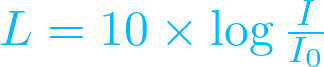• with L in decibel (dB)
• I in W.m-2
• I0 = 10-12 W.m-2

The relationship between the sound intensity and the sound level in decibel is therefore governed by a logarithmic law:

• If we double the sound intensity, the sound intensity level is not doubled. Indeed: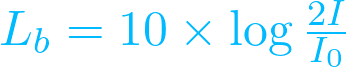so according to the operating rules of logarithms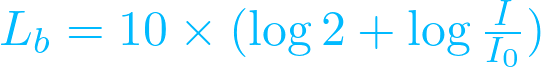from which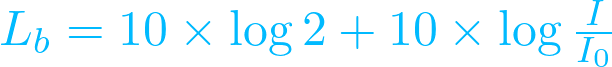therefore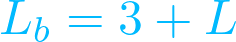Consequently, doubling the sound intensity is equivalent to increasing the sound level by 3 decibels

• The same applies if we divide the sound intensity by two, the sound intensity level decreases by 3 decibels.

## What is the sound frequency?

The vibrations of a sound wave per second are called "sound frequency". Hertz (Hz) is used as the SI unit of frequency. Higher tone frequencies, unlike lower tones, have higher hertz numbers.

## What is sound pressure?

Every sound source emits sound waves, which create waves of high and low pressure in the air. These air pressure fluctuations cause the human eardrum to vibrate, which causes the sound to be heard by the person. How loud a person perceives the emitted sound waves is individual and thus not objectively measurable.

However, the pressure fluctuations generated due to sound waves can be measured physically. Pressure is measured in pascals. Based on the generated sound pressure, the unit decibel can be derived.

### WHAT ARE SOUND WAVES?

The intensitiy of a sound wave is a longitudinal wave that cause us to hear sounds. They propagate from their sound source (e.g., in the form of pressure waves). Humans can hear sounds in a frequency range of 16 to 20,000 Hz. Frequencies below 20 Hz are usually not really heard but rather felt.

### WHAT IS THE SOUND PRESSURE LEVEL?

The sound pressure level is the measure of loudness. The human ear can perceive a sound pressure range from 0.00002 Pascal (Pa) to about 20 Pa. The latter is already the upper hearing limit, also called the "pain limit" due to the high sound pressure. The smallest just audible sound pressure is a tone with a volume of 1 kHz.The following formula can be used to calculate the sound pressure:

Sound level L = 20 x log10(p/p0)

p: Effective sound pressure [Pa]

p0: Reference sound pressure (p0 = 0.00002 Pa)

### SOUND LEVEL AND SOUND INTENSITY

Sounds can have the same sound level but a different frequency. In such cases, these tones are also perceived as being of different volumes. Therefore, in addition to sound pressure and sound pressure level, sound intensity must also be measurable as a purely subjective quantity. The unit of measurement for this is the phon (or fon). This unit of measurement refers to the sound intensity of 10-16 W/c2 at a frequency of 1,000 hertz. This corresponds to a sound pressure level of 2 x 10-10 bar.

1 phon = 10 lg (I1/10-16 W/c2)

Different sound intensities in phon

 Sound Phon Rustling leaves 10 Whispering 20 Normal conversation 50 Street noise 70 Motorcycle 100 Aircraft enginge 120

Different sound intensities in sone

 Phon Sone Description 40 1 Quiet conversation 50 2 Normal conversation 100 64 Loud music (concert)

The psychoacoustic unit of measurement for the subjective loudness N of a sound event is the sone. At 1000 Hz sine, one sone corresponds to 40 phons or 40 decibels.

## How is sound intensity measured?

The intensity of sound is measured in decibel with a sound level meter or Sound pressure Level (SPL) Meter.
The measure of sound intensity is the sound pressure level. The unit forsound intensity is decibels.
A sound intensity level meter consists of a microphone with a preamplifier for measurement, an evaluation unit and a digital display or, in older models, an analog display. Via the measuring microphone, the sound pressure is converted into an analog electrical voltage, via the preamplifier this voltage is then brought into the working range of the detector and the display.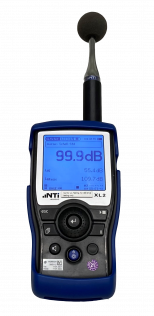Sound level meter

## What is decibel (dB)?

The unit of intensity of sound is decibel (dB). It is used to calculate sound intensity and other physical quantities. Decibel is a tenth of the auxiliary unit of measurement Bel, which was named after the inventor Alexander Graham Bell. The value expressed in decibels is the sound pressure level or sound level. The sound pressure level is abbreviated with Lp (Level Pressure), decibel with the letters dB. To calcualte the sound intensity in decibel, a decibel meter is used. It is a measuring instrument, that assesses sound levels by measuring the sound pressure.

### HOW TO CALCULATE SOUND PRESSURE IN DECIBEL

To calculate decibels of a sound source, two basic values are first required:

• The value of the hearing threshold
• The sound pressure of the noise source

The human hearing threshold is 20 micropascals. The hearing threshold is that value which just produces an audible sound, such as the impact of a spring on the ground. So if the ambient pressure varies by at least 20 micropascals, we can hear something.

To calculate the decibels of a noise source, we need to relate the hearing threshold and the sound pressure of the noise source. The reference point for the sound pressure level in decibels (dB SPL) is the lowest sound pressure that the human ear can hear.

The power of ten is then determined from the result and the ratio is squared. As a result, we get a value in Bel. The tenth of this value is the sound pressure level, expressed in decibels.

Maximum sound pressure level of different sounds in comparison

Whispering has a higher sound pressure level than a dripping faucet. Whispering has a volume level between 30 and 40 dB, while a dripping faucet has a volume level of 20 dB. A quiet radio is about as loud as a moderate rain shower, and a normal conversation, with a volume level of up to 69 dB, is not that far from the sound pressure level of a vacuum cleaner, 70-80dB. The volume of the jackhammer, for example, is exceeded by that of a rock concert (110-120dB).### DOES TWICE AS MUCH DECIBEL ALSO MEAN TWICE AS LOUD?

An increase in sound pressure of 3 dB means a doubling of sound energy, but only an increase of 10 dB is perceived by the human ear as a doubling of volume. Hearing perception thus functions logarithmically.

In addition to the absolute loudness of a signal, the distance between the sound emitter and the human being also influences the perceived loudness. When the distance is doubled, the sound pressure decreases by 6 dB. The signal is then perceived to be approximately a quarter quieter. Use the following range table as a basis for estimating the sound intensity level.

However, environmental factors such as wind speed, wind direction, humidity and weather conditions also play a role in acoustic signals, which is why these are guide values.Audible signaling equipment from Auer Signal is in the range between 60 dB and 127 dB.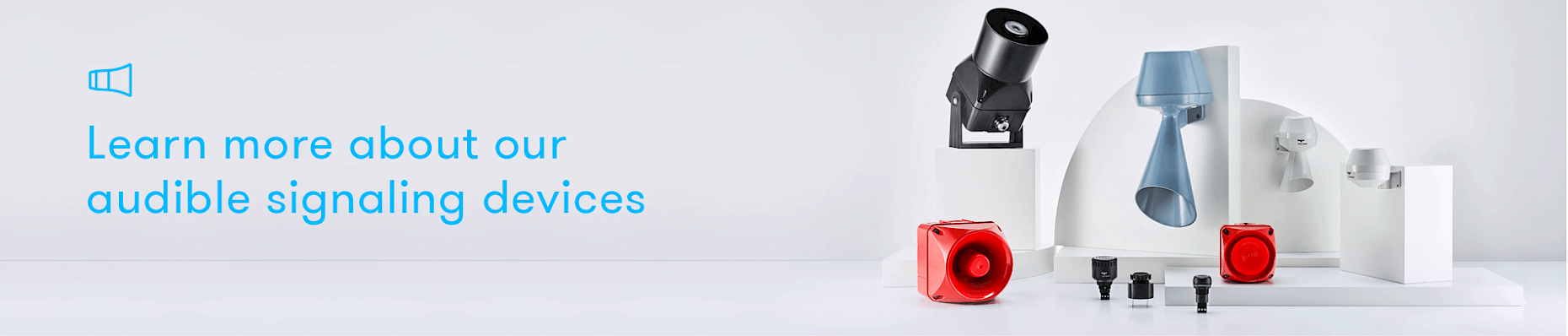## Further technical information:

### Product standards and markings

You can change your settings and withdraw your consent at any time. The lawfulness of the processing carried out on the basis of the consent up to the revocation is not affected by this.